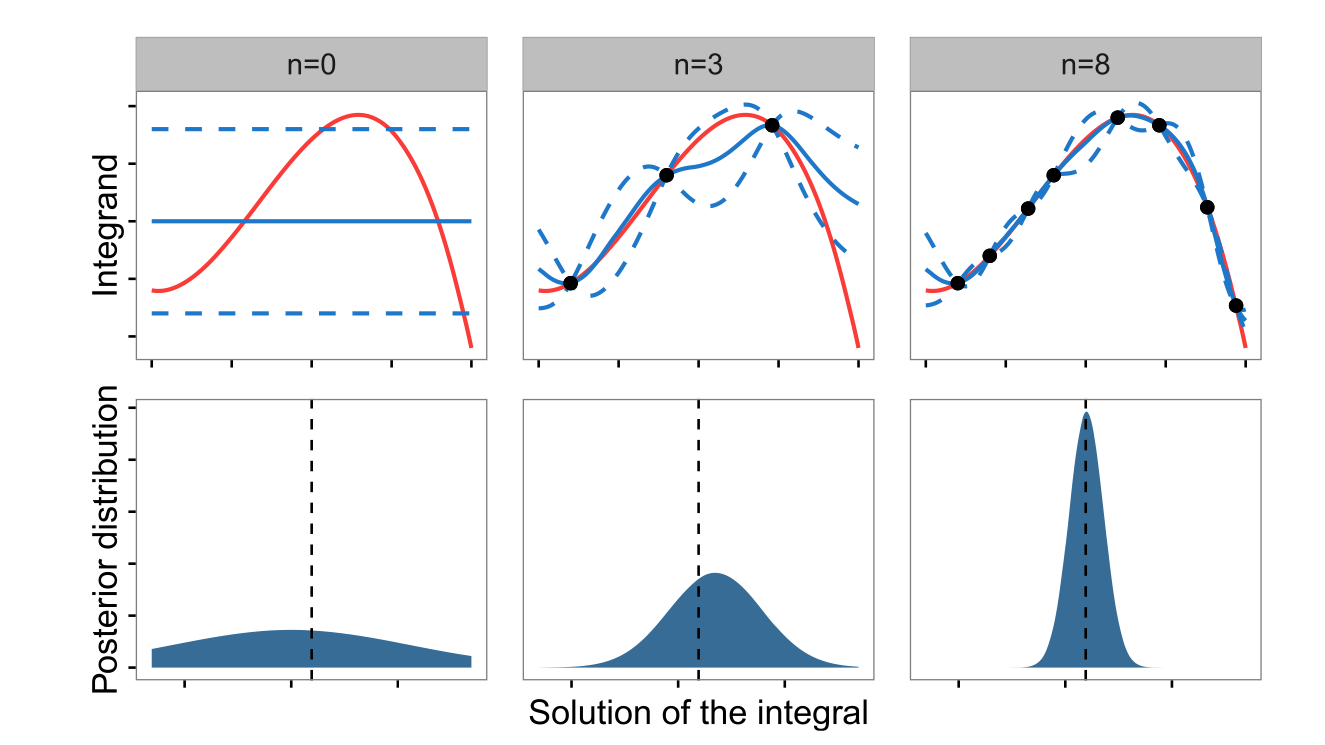# Bayesian Numerical Methods

Whether for computing an integral, solving an optimisation problem, or solving differential equations, numerical methods are one of the core tools of statistics and machine learning. Most of the numerical methods available come from the field of numerical analysis, where one of the common assumption is that a high level of accuracy can be obtain by for example obtaining more function values or using a finer mesh or step-size. In this setting, strong theoretical guarantees can usually be provided in the form of worst-case errors.

Unfortunately, due to the increasing complexity and computational cost of modern statistical machine learning models, there are many application areas where obtaining more function values is simply not feasible. The worst-case theoretical guarantees are therefore not particularly useful since they are designed with an alternative setting in mind, and as a result can be overly pessimistic.

Bayesian numerical methods (sometimes also called probabilistic numerics) provide an alternative way forward through the lense of Bayesian inference. The idea is to consider the solution of the numerical problem as a quantity of interest, then use Bayesian inference to obtain an estimate of this quantity. This allows for the use of prior information to be incorporated in the numerical method (very often using Gaussian processes), and for a detailed description of our uncertainty through the resulting posterior distribution. In fact, these algorithms are particularly useful for statistical applications since they allow for both the inference and the numerics to speak the same language, and as such uncertainty can be propagated throughout the entire procedure.

The most well-know algorithm from this field is Bayesian optimisation, which has been used in academia and industry to tune large scale models. However, there is an increasingly active research field exploring other uses of Bayesian inference for integration, linear algebra or differential equations.## Contributions to this field

My own work has focused on Bayesian quadrature, a family of Bayesian inference schemes for tackling the computation of intractable integrals. Although the first Bayesian quadrature algorithm appeared in the 1980s (and certain aspects even in the 1960s), initial developments were slowed down because computers were not yet able to handle such models. However, the field has recently received renewed interest due to the many advantages provided by Bayesian numerical methods and the rapid improvements in our computing abilities. My own contributions have been on the methodology side, where I have shown how to improve the algorithms for improved computational accuracy, and the theoretical side, where I have provided very general convergence guarantees under a wide range of scenarios including when the Bayesian model is misspecified.

The following paper, which was published with discussion and rejoinder in the journal Statistical Science, is a good starting point for anyone interested in finding out more about this research field:

• Briol, F-X., Oates, C. J., Girolami, M., Osborne, M. A. & Sejdinovic, D. (2019). Probabilistic integration: a role in statistical computation? Statistical Science, Vol 34, Number 1, 1-22. (Journal) (Preprint) (Supplement)

I have also contributed a number of novel algorithms to improve the scalability and accuracy of the method. For example, one of the main questions from a computational viewpoint is where to evaluate the integrand to obtain the best accuracy and uncertainty quantification. Two separate approaches can be found in the papers below:

• Briol, F-X., Oates, C. J., Girolami, M. & Osborne, M. A. (2015). Frank-Wolfe Bayesian Quadrature: probabilistic integration with theoretical guarantees. Advances In Neural Information Processing Systems (NIPS), 1162-1170. (Preprint) (Conference)

• Briol, F-X., Oates, C. J., Cockayne, J., Chen, W. Y. & Girolami, M. (2017). On the sampling problem for kernel quadrature. Proceedings of the 34th International Conference on Machine Learning, PMLR 70:586-595. (Conference) (Preprint)

On the modelling side, I have also extended the approach to use alternatives to standard Gaussian process regression, including through the use of tree-based models, Dirichlet processes and multi-output Gaussian processes:

• Oates, C. J., Niederer, S., Lee, A., Briol, F-X. & Girolami, M. (2017). Probabilistic models for integration error in the assessment of functional cardiac models. Advances in Neural Information Processing Systems (NeurIPS), 109-117. (Conference) (Preprint)

• Xi, X., Briol, F-X. & Girolami, M. (2018). Bayesian quadrature for multiple related integrals. International Conference on Machine Learning, PMLR 80:5369-5378. (Conference) (Preprint)

• Zhu, H., Liu, X., Kang, R., Shen, Z., Flaxman, S., Briol, F-X. (2020). Bayesian probabilistic numerical integration with tree-based models. Neural Information Processing Systems, 5837-5849. (Conference) (Preprint) (Code)

Finally, an interesting by-product of my interest in Bayesian numerical methods has been the following paper, which provides some of the most general theoretical results for Gaussian process regression (including implications for Bayesian optimisation and Bayesian quadrature):

• Wynne, G., Briol, F-X., Girolami, M. (2021). Convergence guarantees for Gaussian process means with misspecified likelihoods and smoothness, Journal of Machine Learning Research, 22 (123), 1-40. (Journal) (Preprint)